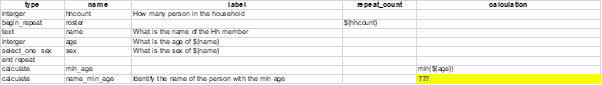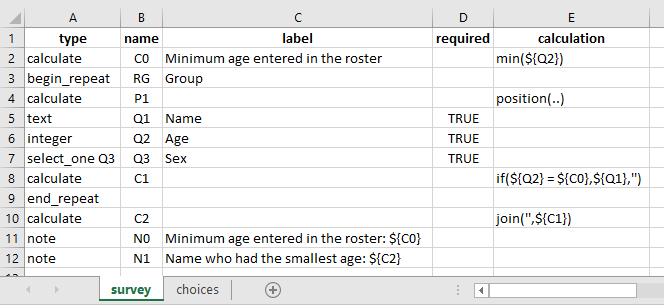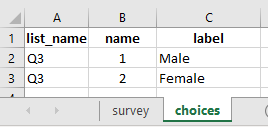# Get the position of a record in a roster based on specific value

Dear all,
I’m working on a roster and I want to have the position number of person in a roster based on a calculated value. In the following example, I want to display the name or the position of the person with minimum age in a roster.
Thanks

type name label repeat_count calculation
interger hhcount How many person in the household
begin_repeat roster \${hhcount}
text name What is the name of the Hh member
interger age What is the age of \${name}
select_one sex sex What is the sex of \${name}
end repeat
calculate min_age min(\${age})
calculate name_min_age Identify the name of the person with the min age ???Welcome to the community, @atchez! Do you have a fixed iteration for this or is it infinite?

@Kal_Lam- I updated the form. The iteration is given by the first question hhcount.

1 Like

The solution is below provided by Jane from ONA.
Thanks and regards

type name label repeat_count calculation
integer hhcount How many person in the household
begin_repeat roster \${hhcount}
text name What is the name of the Hh member
integer age What is the age of \${name}
select_one sex sex What is the sex of \${name}
end repeat
calculate min_age min(\${age})
begin repeat check_youngest \${hhcount}
calculate pos_check position(…)
calculate age_comp indexed-repeat(\${age}, \${roster}, position(…))
calculate name_comp indexed-repeat(\${name}, \${roster},position(…))
calculate display_comp if(\${age_comp} = \${min_age}, \${name_comp}, )
end repeat
calculate selected_name join(’ ',\${display_comp})
note note_name Name selected: \${selected_name}
1 Like

Thank you for sharing the solution with the entire community and making the community rich with resources @atchez! Here is the xlsform for those who wish to try out:

Repeat (Extracting Position).xlsx (11.0 KB)

Here is also a much concise version if you wish to try it out:

#### In the survey tab of your xlsform:#### In the choices tab of your xlsform:#### Reference xlsform:

Repeat Group.xlsx (11.5 KB)